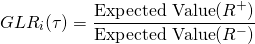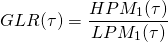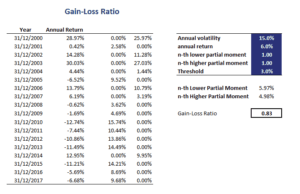# Gain-Loss Ratio (GLR)

The Gain-Loss Ratio (GLR) or Bernardo and Ledoit ratio was introduced by Bernardo and Ledoit (2000). The GLR is an alternative to the Sharpe ratio. The GLR is a downside risk measure similar to the Omega ratio, Sortino ratio, and the Kappa ratio The GLR compares the expected value of positive returns to the expected value of negative returns. Positive returns are returns that exceed a certain threshold. Similarly, negative returns are the returns that do not exceed the threshold. Thus the Gain-Loss formula is the following:On this page, we discuss the gain-loss ratio formula, interpret the ratio, and finally implement the Gain-Loss Ratio in Excel. To implement the ratio in practice, we make use of the first-order Lower Partial Moment.

## Gain-loss ratio formula

The GLR divides the first-order higher partial moment of an investment’s returns by the first-order lower partial moment of the portfolio returns. Thus, we need to calculate the first Lower Partial Moment (LPM) and first (HPM) and divide the latter by the former for a given threshold return tau.

More formally, the formula based on partial moments that we use in practice to estimate GLR looks as follows:where we set tau equal to zero. Thus, all we need to do is calculate the 1st Higher Partial Moment and the 1st Lower Partial Moment.

## Gain-loss ratio in Excel

When expressed in terms of Partial Moments, it is pretty easy to calculate the GLR provided we can estimate the Partial moments. Fortunately, it is straightforward to calculate LPM and HPM using an Excel spreadsheet. The Excel spreadsheet at the bottom of this page implements the GLR.## Summary

We discussed the Gain-Loss ratio, which is the ratio of the expected return of positive returns divided by the expected return of negative returns. It is a measure of downside risk similar to the Sortino and Omega ratio and a good alternative to using the Sharpe Ratio. This ratio is commonly used to evaluate hedge fund managers.

### GLR calculator

Want to have an implementation in Excel? Download the Excel file: Gain-Loss Ratio template